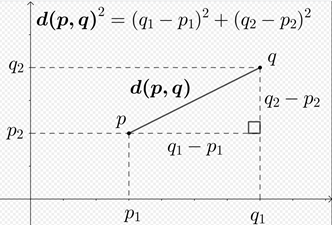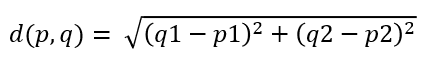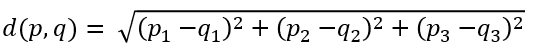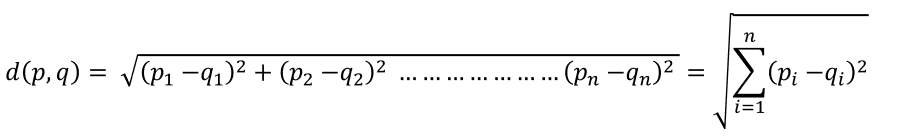# 5 Most Commonly Used Distance Metrics in Machine LearningDifferent types of distance metrics used in Machine Learning. In this article, we will go through 5 of the most commonly used distance metrics.

Whether it is a supervised or unsupervised algorithm, distance metrics play an important role in Machine Learning. Different distance measures are to be chosen depending on the types of data. So it is important that we understand these metrics and how to implement/calculate them. In this article, we will go through 5 of the most commonly used distance metrics.

## 1. Euclidean Distance

Euclidean distance is the straight line distance between two data points in Euclidean space. It is also called as L2 norm or L2 distance.Source: Wikipedia

### Two dimensions

If p=(p1, p2) and q=(q1, q2) are two points in the Euclidean space, the Euclidean distance is given by -### Three dimensions

If p=(p1, p2, p3), q=(q1, q2, q3) are two points in Euclidean space, the Euclidean distance is given by -### n dimensions

If p=(p1, p2…pn) and q=(q1, q2…qn) are two points in Euclidean space, the Euclidean distance is given by -As you might have guessed by now, this formula looks a lot similar to the Pythagoras theorem formula. So, this is also called the Pythagoras Theorem.

## Most popular Data Science and Machine Learning courses — July 2020

Most popular Data Science and Machine Learning courses — August 2020. This list was last updated in August 2020 — and will be updated regularly so as to keep it relevant

## 15 Machine Learning and Data Science Project Ideas with Datasets

Learning is a new fun in the field of Machine Learning and Data Science. In this article, we’ll be discussing 15 machine learning and data science projects.

## Best Free Datasets for Data Science and Machine Learning Projects

This post will help you in finding different websites where you can easily get free Datasets to practice and develop projects in Data Science and Machine Learning.

## 50 Data Science Jobs That Opened Just Last Week

Data Science and Analytics market evolves to adapt to the constantly changing economic and business environments. Our latest survey report suggests that as the overall Data Science and Analytics market evolves to adapt to the constantly changing economic and business environments, data scientists and AI practitioners should be aware of the skills and tools that the broader community is working on. A good grip in these skills will further help data science enthusiasts to get the best jobs that various industries in their data science functions are offering.

## Pipelines in Machine Learning | Data Science | Machine Learning | Python

Machine Learning Pipelines performs a complete workflow with an ordered sequence of the process involved in a Machine Learning task. The Pipelines can also